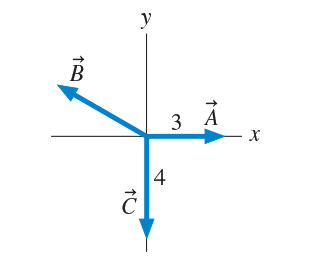# Problem: For the three vectors shown in (Figure 1), A + B + C = 1 ĵ. (Not necessarily to scale.)(a) Write B in component form. Express your answer in terms of the unit vectors î and ĵ.(b) What is the magnitude of B ? Express your answer using two significant figures.(c) What is the direction angle of B , measured clockwise from the negative x axis? Express your answer using two significant figures.

###### FREE Expert Solution

(a) 3 + B - 4 ĵ = 1 ĵ

96% (76 ratings)###### Problem Details

For the three vectors shown in (Figure 1), A + B + C = 1 . (Not necessarily to scale.)(a) Write B in component form. Express your answer in terms of the unit vectors and ĵ.

(b) What is the magnitude of B ? Express your answer using two significant figures.

(c) What is the direction angle of B , measured clockwise from the negative x axis? Express your answer using two significant figures.